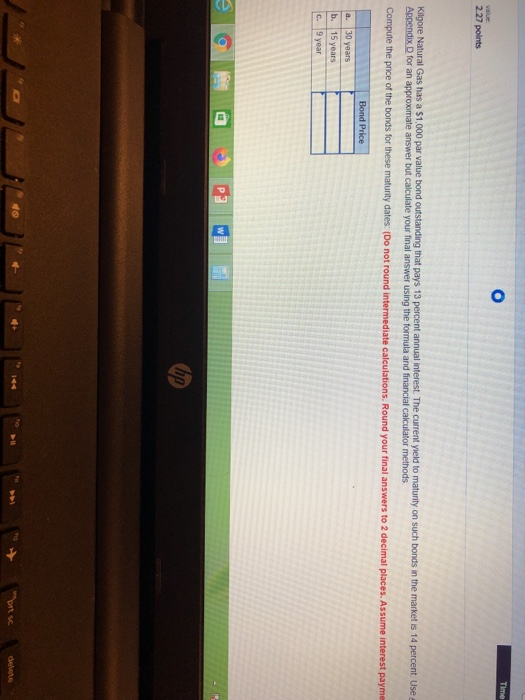# Time value 2.27 points Kilgore Natural Gas has a \$1,000 par value bond outstanding that pays 13 percent annual inte...Time value 2.27 points Kilgore Natural Gas has a \$1,000 par value bond outstanding that pays 13 percent annual interest. The current yield to maturity on such bonds in the market is 14 percent. Use a Appendix D for an approximate answer but calculate your final answer using the formula and financial calculator methods Compute the price of the bonds for these maturity dates: (Do not round intermediate calculations. Round your final answers to 2 decimal places. Assume interest payme Bond Price 30 years a. b. 15 years C 9 year hp prt sc delete 144

A: Using financial calculator
Input: FV= 1000

N=30, PMT=13%*1000=130

I/Y=14, Solve for PV as -929.97

Price of bond = \$929.97

B:

Using financial calculator
Input: FV= 1000

N=15, PMT=13%*1000=130

I/Y=14, Solve for PV as -938.58

Price of bond = \$938.58

C:

Using financial calculator
Input: FV= 1000

N=9, PMT=13%*1000=130

I/Y=14, Solve for PV as -950.54

Price of bond = \$950.54

##### Add Answer of: Time value 2.27 points Kilgore Natural Gas has a \$1,000 par value bond outstanding that pays 13 percent annual inte...
More Homework Help Questions Additional questions in this topic.